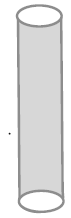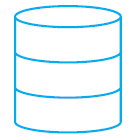# NCERT solutions for class 9 maths chapter 13 Ex 13.2

In this page we have NCERT solutions for class 9 maths chapter 13 for Exercise 13.2 . Hope you like them and do not forget to like , social share and comment at the end of the page.

## class 9 maths chapter 13 Exercise 13.2

We are assuming the value of π=22/7 in all the solutions
Question 1
The curved surface area of a right circular cylinder of height 14 cm is 88 cm2. Find the diameter of the base of the cylinder.
Solution
Height (h) of cylinder = 14 cm
Let the diameter of the cylinder be d.
Curved surface area of cylinder = 88 cm2
2πrh = 88 cm2
r=1 cm
So d=2cm

Question 2
It is required to make a closed cylindrical tank of height 1 m and base diameter 140 cm from a metal sheet. How many square metres of the sheet are required for the same?
Solution
Height of closed cylindrical tank =h=1 m
Base diameter of cylindrical tank =d=140 cm =1.4 m
r= d/2= 0.7 m
Total surface area of cylindrical tank =2π.r(r+h)=2×(22/7)×0.7(0.7+1)
=7.48 m2

Question 3
A metal pipe is 77 cm long. The inner diameter of a cross section is 4 cm, the outer diameter being 4.4 cm . Find its
(i) Inner curved surface area,
(ii) Outer curved surface area,
(iii) Total surface areaSolution
Inner radius of the pipe(a) =2 cm
Outer radius of the pipe(b) =2.2cm
Height of the cylindrical pipe(h)=77 cm
Inner curved area of cylindrical pipe=2 πaH=968 cm2
Outer curved area of cylindrical pipe=2 πbH=1064. cm2
Surface area of whole pipe=Inner curved area + outer curved area +circular area at both ends of the pipe
=2 πaH+2 πbH +2π(b2 –a2)
=2038.08 cm2

Question 4
The diameter of a roller is 84 cm and its length is 120 cm. It takes 500 complete revolutions to move once over to level a playground. Find the area of the playground in m2
Solution
It can be observed that a roller is cylindrical.
Height (h) of cylindrical roller = Length of roller = 120 cm
Radius (r) of the circular end of roller = 84/2=42 cm
Curved surface area of roller = 2πrh
=31680 cm2
Area of field = 500 × CSA of roller
= (500 × 31680) cm2
= 15840000 cm2
= 1584 m2

Question 5
A cylindrical pillar is 50 cm in diameter and 3.5 m in height. Find the cost of painting the curved surface of the pillar at the rate of Rs 12.50 per m2
Solution
Height of the cylindrical pillar =h=3.5 m
Diameter of the cylindrical pillar =d=50 cm
So Radius of the cylindrical pillar =r=50/2=25 cm =0.25 m
So Curved surface of the cylindrical pillar =2π.r.h==5.5 m2
Cost of painting the curved surface of the pillar at the rate of Rs 12.50 per m2
=12.5×5.5= Rs 68.75

Question 6
Curved surface area of a right circular cylinder is 4.4 m2. If the radius of the base of the cylinder is 0.7 m, find its height.
Solution
Let the height of the cylinder be h.
Radius of the base of the cylinder = 0.7 m
Curved surface of cylinder = 4.4m2
2π.r.h=4.4
We know the value of r,so substituting and calculating
h = 1 m

Question 7
The inner diameter of a circular well is 3.5 m. It is 10 m deep. Find
(i) its inner curved surface area,
(ii) the cost of plastering this curved surface at the rate of Rs 40 per m2
Solution
Inner radius (r) of circular well = 1.75 m
Depth (h) of circular well = 10 m
Inner curved surface area = 2πrh
= (44 × 0.25 × 10) m2
= 110 m2
Therefore, the inner curved surface area of the circular well is 110 m2.
Cost of plastering 1 m2 area = Rs 40
So Cost of plastering 100 m2 area = Rs (110 × 40)
= Rs 4400

Question 8
In a hot water heating system, there is a cylindrical pipe of length 28 m and diameter 5 cm. Find the total radiating surface in the system.
Solution
Length of cylindrical pipe =h=28 m
Diameter of cylindrical pipe =d=5 cm
Radius of cylindrical pipe =r=2.5 cm =0.025 m
Total radiating surface in the system=Curved Surface Area of cylindrical pipe =2π.r.h
=2×(22/7)×0.025×28=4.4 m2

Question 9
Find
(i) The lateral or curved surface area of a closed cylindrical petrol storage tank that is  4.2 m in diameter and 4.5 m high.
(ii) How much steel was actually used, if 1/12 of the steel actually used was wasted in making the tank?
Solution
Height of tank=4.5 m
Radius of the circular end of the tank=4.2/2=2.1 m
Lateral curved area of the tank=2π.r.h=59.4 m2
Total surface of tank= Lateral curved area + area of the circular end
=2πr(r+h)=87.12 m2
Let    x  m2  of the sheet is used in making the tank
Then actual sheet in making the tank  = x(1-1/12)=11x/12
Now
11x/12=87.12
Or x=95.04 m2

Question 10
In below figure, you see the frame of a lampshade. It is to be covered with a decorative cloth. The frame has a base diameter of 20 cm and height of 30 cm. A margin of 2.5 cm is to be given for folding it over the top and bottom of the frame. Find how much cloth is required for covering the lampshade.Solution
Height (h) of the frame of lampshade after taking the margin at the top and bottom of the lamppost = (2.5 + 30 + 2.5) cm = 35 cm
Radius (r) of the circular end of the frame of lampshade = 10 cm
Cloth required for covering the lampshade = 2πrh
= 2200 cm2

Question 11
The students of a Vidyalaya were asked to participate in a competition for making and
Decorating penholders in the shape of a cylinder with a base, using cardboard. Each penholder was to be of radius 3 cm and height 10.5 cm. The Vidyalaya was to supply the competitors with cardboard. If there were 35 competitors, how much cardboard was required to be bought for the competition?
Solution
Radius of each pen holder =r=3 cm
Height of each pen holder =h=10.5 cm
Cardboard needed for 1 pen holder = Curved Surface area of 1 pen holder + Area of base of 1 pen holder
=2π.r.h + π.r2
= π.r(2h+r)
=72 π cm2
Cardboard required for 35 pen holders =35 × (22/7)× 3 × 24=7920 cm2

## Summary

1. NCERT solutions for class 9 maths chapter 13 Ex 13.2 has been prepared by Expert with utmost care. If you find any mistake.Please do provide feedback on mail. You can download the solutions as PDF in the below Link also
2. This chapter 13 has total 8 Exercise 13.1 ,13.2 ,13.3,13.4,13.5,13.6,13.7and 13.8. This is the Second exercise in the chapter.